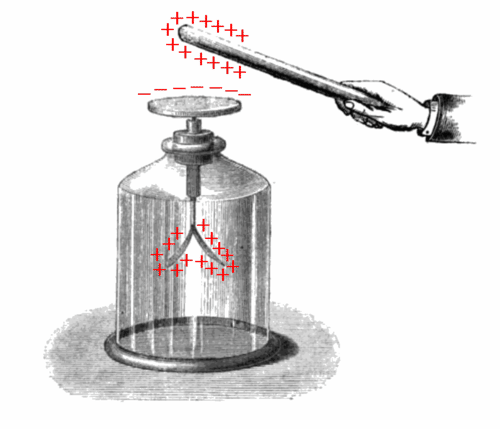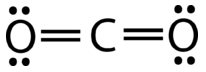# 9.14: Molecular Shapes- No Lone Pairs on Central Atoms

$$\newcommand{\vecs}{\overset { \rightharpoonup} {\mathbf{#1}} }$$ $$\newcommand{\vecd}{\overset{-\!-\!\rightharpoonup}{\vphantom{a}\smash {#1}}}$$$$\newcommand{\id}{\mathrm{id}}$$ $$\newcommand{\Span}{\mathrm{span}}$$ $$\newcommand{\kernel}{\mathrm{null}\,}$$ $$\newcommand{\range}{\mathrm{range}\,}$$ $$\newcommand{\RealPart}{\mathrm{Re}}$$ $$\newcommand{\ImaginaryPart}{\mathrm{Im}}$$ $$\newcommand{\Argument}{\mathrm{Arg}}$$ $$\newcommand{\norm}{\| #1 \|}$$ $$\newcommand{\inner}{\langle #1, #2 \rangle}$$ $$\newcommand{\Span}{\mathrm{span}}$$ $$\newcommand{\id}{\mathrm{id}}$$ $$\newcommand{\Span}{\mathrm{span}}$$ $$\newcommand{\kernel}{\mathrm{null}\,}$$ $$\newcommand{\range}{\mathrm{range}\,}$$ $$\newcommand{\RealPart}{\mathrm{Re}}$$ $$\newcommand{\ImaginaryPart}{\mathrm{Im}}$$ $$\newcommand{\Argument}{\mathrm{Arg}}$$ $$\newcommand{\norm}{\| #1 \|}$$ $$\newcommand{\inner}{\langle #1, #2 \rangle}$$ $$\newcommand{\Span}{\mathrm{span}}$$$$\newcommand{\AA}{\unicode[.8,0]{x212B}}$$Figure $$\PageIndex{1}$$ (Credit: Sylvanus P. Thompson; Source: http://commons.wikimedia.org/wiki/File:Electroscope_showing_induction.png(opens in new window); License: Public Domain)

### How does an electroscope work?

An electroscope is a device used to study charge. When a positively charged object (the rod) nears the upper post, electrons flow to the top of the jar, leaving the two gold leaves positively charged. The leaves repel each other since both hold positive, like charges. The VSEPR theory says that electron pairs, also a set of like charges, will repel each other such that the shape of the molecule will adjust, so that the valence electron pairs stay as far apart from each other as possible.

## Central Atom with No Lone Pairs

In order to easily understand the types of molecules possible, we will use a simple system to identify the parts of any molecule:

• A = central atom in a molecule
• B = atoms surrounding the central atom

Subscripts after the B will denote the number of B atoms that are bonded to the central A atom. For example, AB$$_4$$ is a molecule with a central atom surrounded by four covalently bonded atoms. Again, it does not matter if those bonds are single, double, or triple bonds.

### AB$$_2$$: Beryllium Hydride $$\left( \ce{BeH_2} \right)$$

Beryllium hydride consists of a central beryllium atom with two single bonds to hydrogen atoms. Recall that it violates the octet rule.

$\ce{H-Be-H}\nonumber$

According to the requirement that electron pairs maximize their distance from one another, the two bonding pairs in the $$\ce{BeH_2}$$ molecules will arrange themselves on directly opposite sides of the central $$\ce{Be}$$ atom. The resulting geometry is a linear molecule, shown in the figure below in a "ball and stick" model.Figure $$\PageIndex{2}$$: Beryllium hydride model. (Credit: Ben Mills (User:Benjah-bmm27/Wikimedia Commons); Source: http://commons.wikimedia.org/wiki/File:Beryllium-hydride-molecule-IR-3D-balls.png(opens in new window); License: Public Domain)

The bond angle from $$\ce{H-Be-H}$$ is $$180^\text{o}$$ because of its linear geometry.

Carbon dioxide is another example of a molecule which falls under the AB$$_2$$ category. Its Lewis structure consists of double bonds between the central carbon and the oxygen atoms (see figure below).Figure $$\PageIndex{3}$$: Carbon dioxide bonding. (Credit: Joy Sheng; Source: CK-12 Foundation; License: CC BY-NC 3.0(opens in new window))

The repulsion between the two groups of four electrons (two pairs) is no different than the repulsion of the two groups of two electrons (one pair) in the $$\ce{BeH_2}$$ molecule. Carbon dioxide is also linear (see figure below).Figure $$\PageIndex{4}$$: Carbon dioxide. (Credit: User:Benji9072/Wikimedia Commons; Source: http://commons.wikimedia.org/wiki/File:Carbon_dioxide_structure.png(opens in new window); License: Public Domain)

### AB$$_3$$: Boron Trifluoride $$\left( \ce{BF_3} \right)$$

Boron trifluoride consists of a central boron atom with three single bonds to fluorine atoms (see figure below). The boron atom also has an incomplete octet.Figure $$\PageIndex{5}$$: Boron trifluoride bonding. (Credit: Joy Sheng; Source: CK-12 Foundation; License: CC BY-NC 3.0(opens in new window))

The geometry of the $$\ce{BF_3}$$ molecule is called trigonal planar (figure $$\PageIndex{3}$$). The fluorine atoms are positioned at the vertices of an equilateral triangle. The $$\ce{F-B-F}$$ angle is $$120^\text{o}$$ and all four atoms lie in the same plane.Figure $$\PageIndex{6}$$: Boron trifluoride model. (Credit: User:Benji9072/Wikimedia Commons; Source: http://commons.wikimedia.org/wiki/File:Boron-trifluoride-3D-balls.png(opens in new window); License: Public Domain)

### AB$$_4$$: Methane $$\left( \ce{CH_4} \right)$$

Methane is an organic compound that is the primary component of natural gas. Its structure consists of a central carbon atom with four single bonds to hydrogen atoms (see figure below). In order to maximize their distance from one another, the four groups of bonding electrons do not lie in the same plane. Instead, each of the hydrogen atoms lie at the corners of a geometrical shape called a tetrahedron. The carbon atom is at the center of the tetrahedron. Each face of a tetrahedron is an equilateral triangle.Figure $$\PageIndex{7}$$: Tetrahedral structure of methane. (Credit: (Left) Pearson Scott Foresman; (Right) Ben Mills (Wikimedia: Benjah-bmm27); Source: (Left) http://commons.wikimedia.org/wiki/File:Tetrahedron_(PSF).png(opens in new window); (Right) http://commons.wikimedia.org/wiki/File:Methane-CRC-MW-3D-balls.png(opens in new window); License: Public Domain)

The molecular geometry of the methane molecule is tetrahedral (see figure $$\PageIndex{5}$$). The $$\ce{H-C-H}$$ bond angles are $$109.5^\text{o}$$, which is larger than the $$90^\text{o}$$ that they would be if the molecule was planar. When drawing a structural formula for a molecule such as methane, it is advantageous to be able to indicate the three-dimensional character of its shape. The structural formula below is called a perspective drawing. The dotted line bond is to be visualized as receding into the page, while the solid triangle bond is to be visualized as coming out of the page.Figure $$\PageIndex{8}$$: Methane perspective model. (Credit: Joy Sheng; Source: CK-12 Foundation; License: CC BY-NC 3.0(opens in new window))

## Summary

• Electron pairs repel each other and influence bond angles and molecular shape.

## Review

1. What are the bond angles in carbon dioxide?
2. What molecule has bond angles of 109.5°?
3. What is the geometry of the BF3 molecule?

This page titled 9.14: Molecular Shapes- No Lone Pairs on Central Atoms is shared under a CK-12 license and was authored, remixed, and/or curated by CK-12 Foundation via source content that was edited to the style and standards of the LibreTexts platform; a detailed edit history is available upon request.